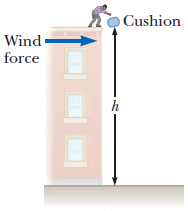# I'm stuck! This is a combination of dynamics and free fall

## Homework Statement

A flat cushion of mass m is released from rest at the corner of the roof of a building, at height h. A wind blowing along the side of the building exerts a constant horizontal force of magnitude F on the cushion as it drops as shown in the figure below. The air exerts no vertical force.

image:If m = 1.03 kg, h = 7.85 m, F = 2.29 N, how far from the building (in m) will the cushion hit the level ground?

F = ma

## The Attempt at a Solution

I tried solving for the acceleration: F = ma ---> 2.29 = (1.03kg)(a) -----> a = 2.22 m/s^2
Then I solved for the time the object hit the ground in the y-direction: d = vit + at^2/2 ----> -7.85 = -9.8/2 t^2
-----> t = 2.22s
My solutions above might just be wrong. That's where I'm stuck right now.

#### Attachments

Baluncore
2021 Award
Then I solved for the time the object hit the ground in the y-direction: d = vit + at^2/2 ----> -7.85 = -9.8/2 t^2
You did not write out the equation before substituting numerical values.
How long will it be in free fall? You know; h = 1/2 ⋅ a ⋅ t2
t2 = 2 ⋅ h / a
t = Sqrt( 2 ⋅ h / a )
t = Sqrt( 2 * 7.85 / 9.8 )
t = 1.265 sec

haruspex
Homework Helper
Gold Member

## Homework Statement

A flat cushion of mass m is released from rest at the corner of the roof of a building, at height h. A wind blowing along the side of the building exerts a constant horizontal force of magnitude F on the cushion as it drops as shown in the figure below. The air exerts no vertical force.
View attachment 231892
A person stands on the roof of a building and releases a cushion from a height h above the ground. A rightward arrow indicates the wind force incident upon the cushion.
If the cushion is thrown downward with a nonzero speed at the top of the building, what will be the shape of its trajectory?

Explain.

## The Attempt at a Solution

I can't exactly put it into words that make sense, but I just imagine that if you throw it at a certain speed downwards, the wind would push it towards the right, and make this parabolic shape. Can someone help me explain it in a clear way?[/B]
•